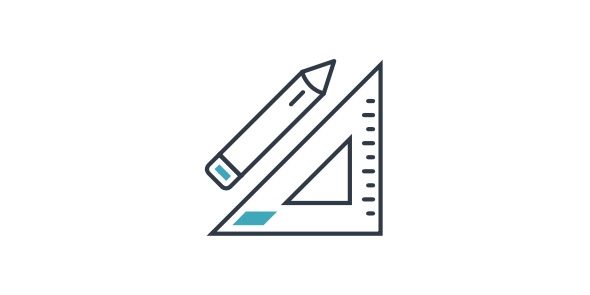# 8 Maths Revision For Term 2 Test.

22 Questions | Total Attempts: 352Settings20 Multiple choice questions. Attempt every questions. Calculators not allowed. Use working out paper during test.

• 1.
Which of the following is an example of an improper fraction?
• A.

3/4

• B.

4/3

• C.

4 1/3

• D.

1/3

• 2.
Which of the following fractions is equivalent to 4/5  (there is more than one correct answer)
• A.

40/50

• B.

8/10

• C.

16/25

• D.

12/15

• 3.
Expressed as a fraction in simplest terms 0.02 is equal to:
• A.

2/10

• B.

2/100

• C.

1/5

• D.

1/50

• 4.
The reciprocal of 3/5 is...
• A.

3

• B.

5/3

• C.

5

• D.

2/5

• 5.
3x + 2x =
• A.

6x

• B.

5x

• C.

￼ 6 + 2x

• D.

6 (x + x)

• 6.
2 x a x 3 x b =
• A.

6ab

• B.

23ab

• C.

2a + 3b

• D.

None of the above

• 7.
2 (a + 3) =
• A.

2a + 3

• B.

A + 6

• C.

2a + 6

• D.

None of the above

• 8.
Solve y for 2y + 3 = 13 is
• A.

Y = 3

• B.

Y = 4

• C.

Y = 5

• D.

Y = 6

• 9.
Simplify 21/30
• A.

2/3

• B.

7/10

• C.

3/10

• 10.
Express 12/5 as a mixed numeral
• A.

5 2/12

• B.

2 2/5

• C.

2.20

• D.

15 2/5

• 11.
Evaluate 3/7 + 2/7
• A.

5/14

• B.

14/21

• C.

5/7

• D.

I don't know how to do this

• 12.
Evaluate 2.83 + 7.29
• A.

10.12

• B.

10.29

• C.

9.12

• 13.
True or False? 0.1212 is a recurring decimal?
• A.

True

• B.

False

• 14.
Simplify 3y + 2 + 2y +5
• A.

5y + 7y

• B.

5y + 7

• C.

12y

• D.

I don't know how to do this

• 15.
7m x 3 =
• A.

7m + 3m

• B.

21m

• C.

21 + m

• 16.
Factorise 3m - 3n
• A.

3m (3 - m)

• B.

3mn

• C.

3m - n

• D.

3 (m - n)

• 17.
Factorise 3z + 21a
• A.

24az

• B.

7az

• C.

3 (z + 7a)

• D.

7 (3a + z)

• 18.
Factorise 2b + 36m
• A.

2(b + 18m)

• B.

B(2 + 36m)

• C.

36(b + m)

• 19.
Expand 4(a + 7b)
• A.

7a + 28b

• B.

28abn

• C.

7abn

• D.

4a + 7b

• 20.
Expand a (16 + 7 - 2b)
• A.

A + b - 2 + 23

• B.

21ab

• C.

16a + 7 - 2b

• D.

23a - 2ab

• 21.
Express 0.6 as a percentage
• A.

6%

• B.

60%

• C.

0.6%

• D.

6.0%

• 22.
Express 2/7 as a percentage to the nearest 2 decimal places.
• A.

27.72 %

• B.

28.57 %

• C.

207.27%

• D.

27.00%

Related TopicsBack to top